# The TRANSREG Procedure

### Example 104.1 Transformation Regression of Exhaust Emissions Data

In this example, the data are from an experiment in which nitrogen oxide emissions from a single cylinder engine are measured for various combinations of fuel, compression ratio, and equivalence ratio. The data are provided by Brinkman (1981). This gas data set is available from the Sashelp library.

The equivalence ratio and nitrogen oxide variables are continuous and numeric, so spline transformations of these variables are requested. The spline transformation of the dependent variable is restricted to be monotonic. Each spline is degree three with nine knots (one at each decile) in order to give PROC TRANSREG a great deal of freedom in finding transformations. The compression ratio variable has only five discrete values, so an optimal scoring is requested with monotonicity constraints. The character variable Fuel is nominal, so it is optimally scored without any monotonicity constraints. Observations with missing values are excluded with the NOMISS a-option.

ods graphics on;

title 'Gasoline Example';
title2 'Iteratively Estimate NOx, CpRatio, EqRatio, and Fuel';

* Fit the Nonparametric Model;
proc transreg data=sashelp.Gas solve test nomiss plots=all;
ods exclude where=(_path_ ? 'MV');
model mspline(NOx / nknots=9) = spline(EqRatio / nknots=9)
monotone(CpRatio) opscore(Fuel);
run;


Output 104.1.1: Transformation Regression Example: The Nonparametric Model

 Gasoline Example Iteratively Estimate NOx, CpRatio, EqRatio, and Fuel

The TRANSREG Procedure

 Dependent Variable Mspline(NOx)Nitrogen Oxide

 Number of Observations Read 171 169

TRANSREG MORALS Algorithm Iteration History for Mspline(NOx)
Iteration
Number
Average
Change
Maximum
Change
R-Square Criterion
Change
Note
0 0.41900 3.80550 0.05241
1 0.11984 0.83327 0.91028 0.85787
2 0.03727 0.17688 0.93981 0.02953
3 0.02795 0.10880 0.94969 0.00987
4 0.02088 0.07279 0.95382 0.00413
5 0.01530 0.05031 0.95582 0.00201
6 0.01130 0.03922 0.95688 0.00106
7 0.00852 0.03197 0.95748 0.00060
8 0.00657 0.02531 0.95783 0.00035
9 0.00510 0.01975 0.95805 0.00022
10 0.00398 0.01534 0.95818 0.00013
11 0.00314 0.01200 0.95827 0.00009
12 0.00250 0.00953 0.95832 0.00005
13 0.00199 0.00752 0.95836 0.00003
14 0.00159 0.00594 0.95838 0.00002
15 0.00127 0.00470 0.95839 0.00001
16 0.00102 0.00373 0.95840 0.00001
17 0.00081 0.00297 0.95841 0.00001
18 0.00065 0.00237 0.95841 0.00000
19 0.00052 0.00189 0.95841 0.00000
20 0.00042 0.00151 0.95842 0.00000
21 0.00033 0.00120 0.95842 0.00000
22 0.00027 0.00096 0.95842 0.00000
23 0.00021 0.00077 0.95842 0.00000
24 0.00017 0.00061 0.95842 0.00000
25 0.00014 0.00049 0.95842 0.00000
26 0.00011 0.00039 0.95842 0.00000
27 0.00009 0.00031 0.95842 0.00000
28 0.00007 0.00025 0.95842 0.00000
29 0.00006 0.00020 0.95842 0.00000
30 0.00005 0.00016 0.95842 0.00000 Not Converged

 WARNING: Failed to converge, however criterion change is less than 0.0001.

 The TRANSREG Procedure Hypothesis Tests for Mspline(NOx)Nitrogen Oxide

Univariate ANOVA Table Based on the Usual Degrees of Freedom
Source DF Sum of Squares Mean Square F Value Liberal p
Model 21 326.0176 15.52465 161.35 >= <.0001
Error 147 14.1443 0.09622
Corrected Total 168 340.1619

 Root MSE R-Square 0.31019 0.9584 2.34593 0.9525 13.2226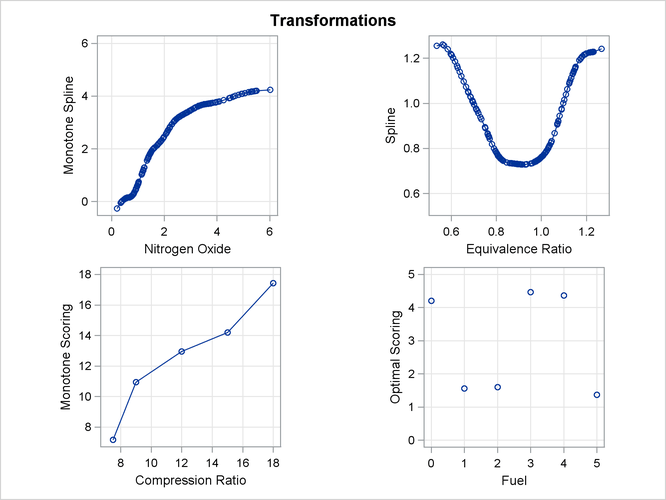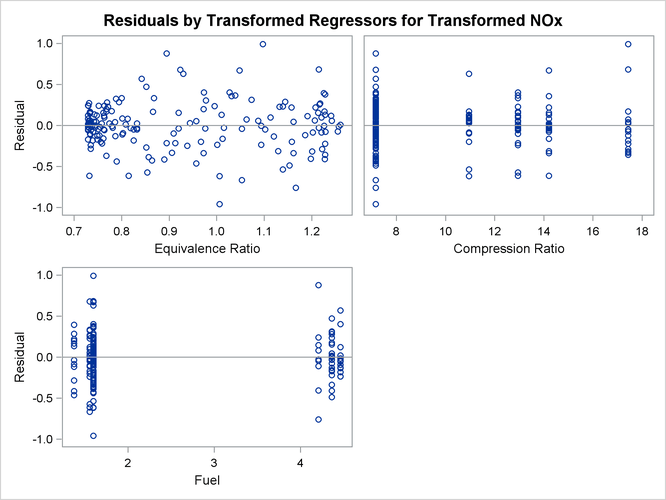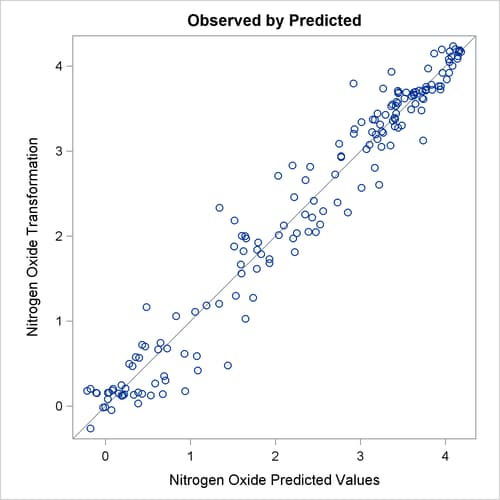The squared multiple correlation for the initial model is approximately 0.05. PROC TRANSREG increases the R square to over 0.95 by transforming the variables. The transformation plots show how each variable is transformed. The transformation of compression ratio (TCpRatio) is nearly linear. The transformation of equivalence ratio (TEqRatio) is nearly parabolic. It can be seen from this plot that the optimal transformation of equivalence ratio is nearly uncorrelated with the original scoring. This suggests that the large increase in R square is due to this transformation. The transformation of nitrogen oxide (TNOx) is similar to a log transformation. The final plot shows the transformed dependent variable plotted as a function of the predicted values. This plot is reasonably linear, showing that the nonlinearities in the data are being accounted for fairly well by the TRANSREG model.

These results suggest the parametric model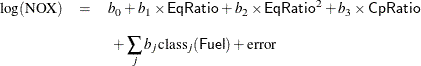You can perform this analysis with PROC TRANSREG. The following statements produce Output 104.1.2:

title2 'Now fit log(NOx) = b0 + b1*EqRatio + b2*EqRatio**2 +';
title3 'b3*CpRatio + Sum b(j)*Fuel(j) + Error';

*-Fit the Parametric Model Suggested by the Nonparametric Analysis-;
proc transreg data=sashelp.Gas solve ss2 short nomiss plots=all;
model log(NOx) = pspline(EqRatio / deg=2) identity(CpRatio)
opscore(Fuel);
run;


Output 104.1.2: Transformation Regression Example: The Parametric Model

 Gasoline Example Now fit log(NOx) = b0 + b1*EqRatio + b2*EqRatio**2 + b3*CpRatio + Sum b(j)*Fuel(j) + Error

The TRANSREG Procedure

 Dependent Variable Log(NOx)Nitrogen Oxide

 Number of Observations Read 171 169

Log(NOx)
Algorithm converged.

 The TRANSREG Procedure Hypothesis Tests for Log(NOx)Nitrogen Oxide

Univariate ANOVA Table Based on the Usual Degrees of Freedom
Source DF Sum of Squares Mean Square F Value Pr > F
Model 8 79.33838 9.917298 213.09 <.0001
Error 160 7.44659 0.046541
Corrected Total 168 86.78498

 Root MSE R-Square 0.21573 0.9142 0.6313 0.9099 34.1729

Univariate Regression Table Based on the Usual Degrees of Freedom
Variable DF Coefficient Type II
Sum of
Squares
Mean Square F Value Pr > F Label
Intercept 1 -15.274649 57.1338 57.1338 1227.60 <.0001 Intercept
Pspline.EqRatio_1 1 35.102914 62.7478 62.7478 1348.22 <.0001 Equivalence Ratio 1
Pspline.EqRatio_2 1 -19.386468 64.6430 64.6430 1388.94 <.0001 Equivalence Ratio 2
Identity(CpRatio) 1 0.032058 1.4445 1.4445 31.04 <.0001 Compression Ratio
Opscore(Fuel) 5 0.158388 5.5619 1.1124 23.90 <.0001 Fuel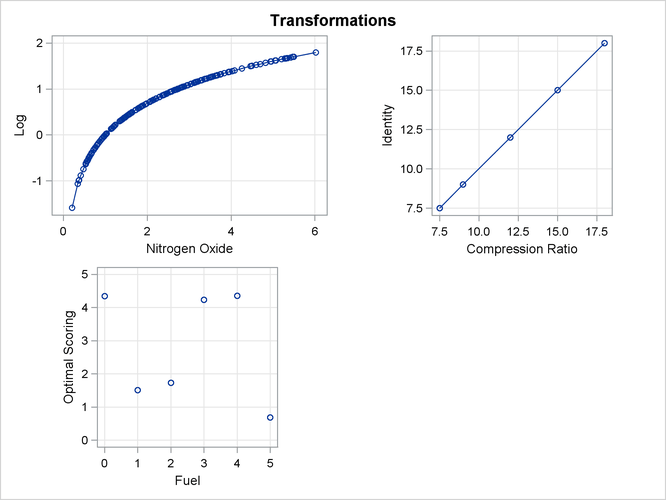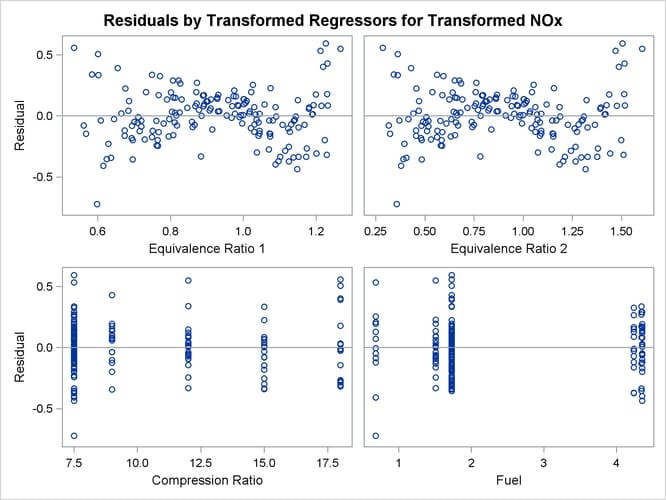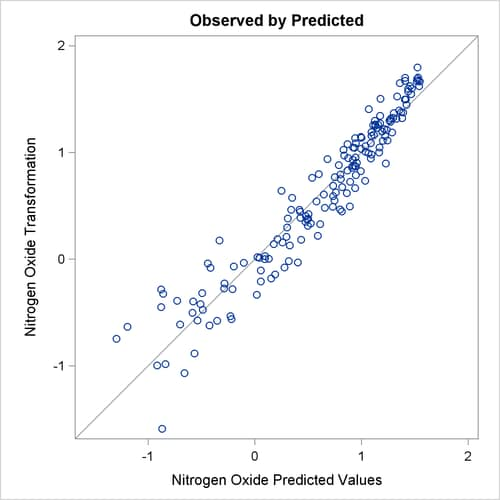The LOG transformation computes the natural log. The PSPLINE expansion expands EqRatio into a linear term, EqRatio, and a squared term,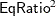. An identity transformation of CpRatio and an optimal scoring of Fuel is requested. These should provide a good parametric operationalization of the optimal transformations. The final model has an R square of 0.91 (smaller than before since the model has fewer parameters, but still quite good).